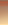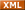# 李泽然---路在自己脚下！***只有当潮水退去的时候，才知道谁在裸泳***C++博客 联系 聚合管理/** Name: IP Address* Funcion: To convert binary numbers to decimal numbers* Input: 00000011100000001111111111111111* Output: 3.128.255.255*/#include
<stdio.h>void main(){char input;int output;int count = -1;int i;for(i = 0; i < 4; i++ )output[i]
= 0;gets( input );for( i = 0; i < 32; i++ ){if( i % 8 == 0 )count
++;if( input[i] == '1' ){switch( i % 8 ){case 0:output[count]
+= 128;break;case 1:output[count]
+= 64;break;case 2:output[count]
+= 32;break;case 3:output[count]
+= 16;break;case 4:output[count]
+= 8;break;case 5:output[count]
+= 4;break;case 6:output[count]
+= 2;break;case 7:output[count]
+= 1;break;default:break;}}}printf(
"%d.%d.%d.%d\n", output, output, output, output );}
posted on 2008-12-31 21:44 lzr 阅读(505) 评论(1)  编辑 收藏 引用 所属分类: C/C++### Feedback

# re: 将二进制IP地址转换成十进制 2009-01-01 14:24 jbt.zh